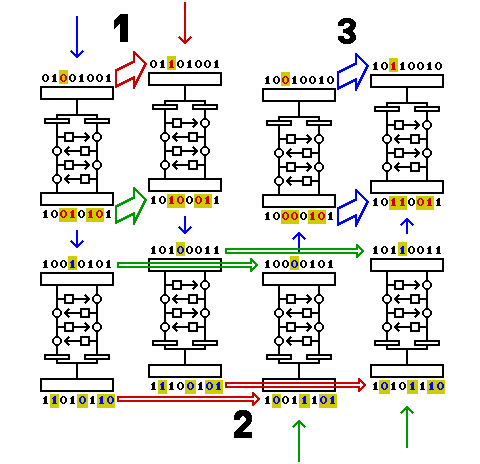[Next] [Up/Previous] [Index]

# Extensions of Differential Cryptanalysis

The technique of differential cryptanalysis, in addition to being very powerful by itself, has served as a basis for the development of even more powerful techniques, such as those surveyed here and in the next section.

### Truncated differentials

It is of course possible that some of the bits of E(A,k) xor E(B,k) will be more likely to match those of Y than others. If one can, in addition, ignore some of the bits of A and B, one has a truncated differential for the cipher being attacked, and this technique, due to Lars R. Knudsen, has been found to be very powerful. (Being able to ignore some bits of A and B may allow two or more truncated differentials to be used together, and this is why it is important.)

### Higher-order Differentials

Another important addition to the available techniques deriving from differential cryptanalysis is the use of higher-order differentials, which first appeared in a paper by Xuejia Lai.

A differential characteristic of the type described above, where for a large number of different values of A, B equals A xor X, and the encrypted versions of A and B for a given key, k, are expected to have the relation E(A,k) = E(B,k) xor Y, if a target statement about the key k is true, can be made analogous to a derivative in calculus, and then it is termed that Y is the first derivative of the cipher E at the point X.

A second-order derivative would then be one involving a second quantity, W, such that E(A,k) xor E(B,k) = E(C,k) xor E(D,k) xor Z is expected to be true more often than would be true due to chance, where not only is B = A xor X, but C = A xor W and D = B xor W. In that case, Z is the second derivative of the cipher E at the point X,W. Since xor performs the function of addition and subtraction, the four items encrypted for any A are just lumped together in this case, but if differential cryptanalysis were being performed over another field where the distinction is significant, then Y=E(A+X,k)-E(A,k) and Z=(E(A+X+W,k)-E(A+W,k))-(E(A+X,k)-E(A,k)) would be the appropriate equations to use. This technique is important because a second order derivative can exist at a point for the first coordinate of which no first order derivative exists, or is probable enough to be useful.

And similarly, a third order derivative is derived from the difference of two second order derivatives, based on another constant difference, and so on.

### The Boomerang Attack

Recently, a means of improving the flexibility of differential cryptanalysis was discovered by David A. Wagner. Called the boomerang attack, it allows the use of two unrelated characteristics for attacking two halves of a block cipher.This diagram shows how the attack might work if everything goes perfectly for a particular initial block. The numbered points in the diagram show the steps involved in the attack.

1. Start with a random block of plaintext. Based on the characteristic known for the first half of the cipher, if we XOR a certain vector with it, called d1 (equal to 00100000 in the diagram), the result after half-enciphering the two plaintext blocks, before and after the XOR, will differ by c1 (equal to 00110110 in the diagram), if what we wish to learn about the key happens to be true.
2. Since the characteristic applies only to the first half of the cipher, the results after the whole block cipher won't be related. Take those two results, and XOR each one with d2 (equal to 01001011 in the diagram), which is the vector corresponding to the characteristic for the second half of the cipher. In each case, XORing d2 with a ciphertext block is expected to change the result after deciphering halfway by c2 (equal to 00010000 in the diagram), again, if something is true of the key.
3. With two intermediate results that differ by c1, if each one has c2 XORed to it, the two results of the XOR will still differ by c1. Since this difference now relates to the first half characteristic, it can be seen in the final output, thus indicating the truth or otherwise of two hypotheses about the key.

This increases the potential effectiveness of differential cryptanalysis, because one can make use of characteristics that do not propagate through the complete cipher. Also, certain kinds of added complexities, such as a bit transpose in the middle of the cipher, do not serve as a barrier to this method, since two values differing by an XOR with some value merely differ by an XOR with some other value after a bit transpose.

However, it has its limitations. It only produces a result if both characteristics are present; it does not allow testing for each characteristic independently. Even so, it seems to double the number of rounds a cipher needs to be considered secure.

Since at one end of a sequence of rounds, the precise difference between blocks that is required for the characteristic must be input, it isn't possible directly to cascade this method to break a block cipher into four or more pieces.

Note that any single Feistel round has a large family of "characteristics" that is 100% probable, but which tells nothing about the key, since any pattern that involves leaving the half that is input to the F-function unchanged, but involves an XOR to the half that is XORed with the output of the F-function applies, so one of the things this method can do is allow the use of attacks against the first or last 15 rounds of DES against 16-round DES. Hence, if by some other trick a block cipher with 16 rounds could be broken into 16 pieces like this, one could test for an informative characteristic which applied to any single round.

[Next] [Up/Previous] [Index]